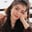Related Tags

dataframe
mod
communitycreator
pandas

# How to use the DataFrame.mod() method in pandasFouzia Bashir

### Overview

The DataFrame.mod() method from the pandas module is used to calculate the element-wise modulus (binary operator mod) of two DataFrames, Series, or Constants.

Note: We can also modulo operator % instead. However, it does not deal with empty NaN valuesfields.

### Syntax

DataFrame.mod(other, axis='columns', level=None, fill_value=None)
DataFrame.mod() Syntax

### Parameters

It takes the following argument values:

• other: This can be a DataFrame, Series, or Constant.
• axis: This can be 0 or index or 1 or columns. Its default value is 'columns'.
• level: The broadcast value across a level can either be int or None. Its default value is None.
• fill_value: This can either be a float value or None. Its default value is None.

### Return value

It returns a DataFrame containing modulo results.

### Explanation

In the code snippet below, we'll calculate the modulus of:

1. DataFrame objectCallee object and argument constant value.
2. DataFrame object and argument DataFrame object.
3. DataFrame object and argument Series object.

# importing Pandas and Numpy
import pandas as pd
import numpy as np
# Creating a DataFrame
df1= pd.DataFrame([[5, 3, 6, 4],
[11, None, 4, 3],
[4, 3, 8, None],
[5, 4, 2, 8]])
# Creating another DataFrame
df2= pd.DataFrame([[None, 4, 5, 9],
[1, None, 4, 3],
[14, 3, -1, None],
[2, 14, 8, 8]])
# Creating Numpy array
data = np.array([6, 12, -3, 9])
# Converting to Python series
series = pd.Series(data)

"""DataFrame and Constant"""
# Invoking mod() method
# Evaluating modulus between df1 and constant
modulus= df1.mod(3, fill_value = 2)
# print results
print("DataFrame and Constant")
print(modulus)

"""Both are DataFrames"""
# Evaluating modulus between df1 and df2
modulus= df1.mod(df2, fill_value = 2)
print("\nBoth are DataFrames")
print(modulus)

"""DataFrame and Series"""
# Evaluating modulus between df1 and series
modulus= df1.mod(series)
print("\nDataFrame and Series")
print(modulus)

Different usecases of DataFrame mod()

### Explanation

• Lines 5 to 13: We create two numeric DataFrames, each containing four rows and four columns.
• Lines 15 to 17: We create a pandas series of 4 values.
• Line 22: We calculate the modulus of df1 object and the argument constant value.
• Line 29: We calculate the modulus of df1 object and the argument DataFrame df2 object.
• Line 35: We calculate the modulus of df1 object and the argument series object.

RELATED TAGS

dataframe
mod
communitycreator
pandas

CONTRIBUTORFouzia Bashir
RELATED COURSES

View all Courses

Keep Exploring

Learn in-demand tech skills in half the time